Thermodynamics Thermodynamics is the study of energy and

• Slides: 24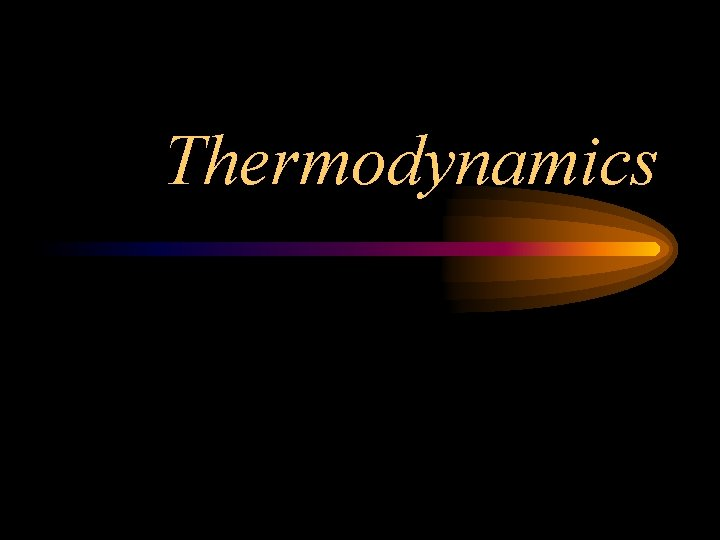ThermodynamicsThermodynamics is the study of energy and its transformations. Thermodynamics is based upon a series of laws.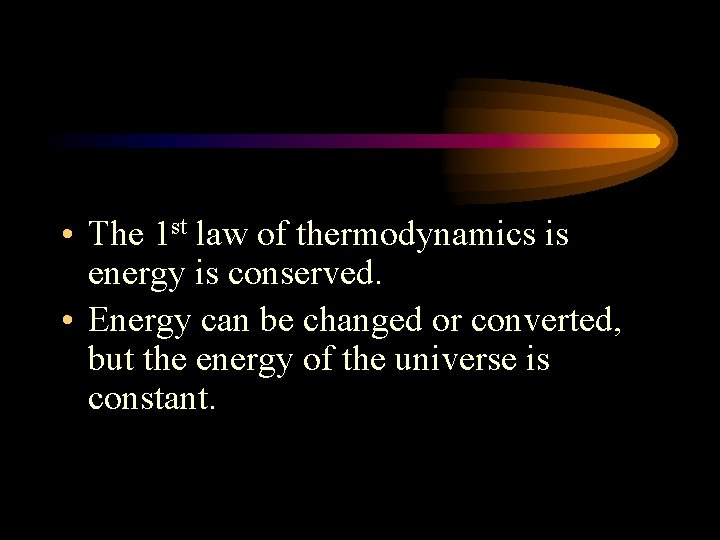• The 1 st law of thermodynamics is energy is conserved. • Energy can be changed or converted, but the energy of the universe is constant.There are two divisions of energy: 1. Kinetic 2. Potential • Kinetic energy is energy in use or motion. • Potential energy is stored energy.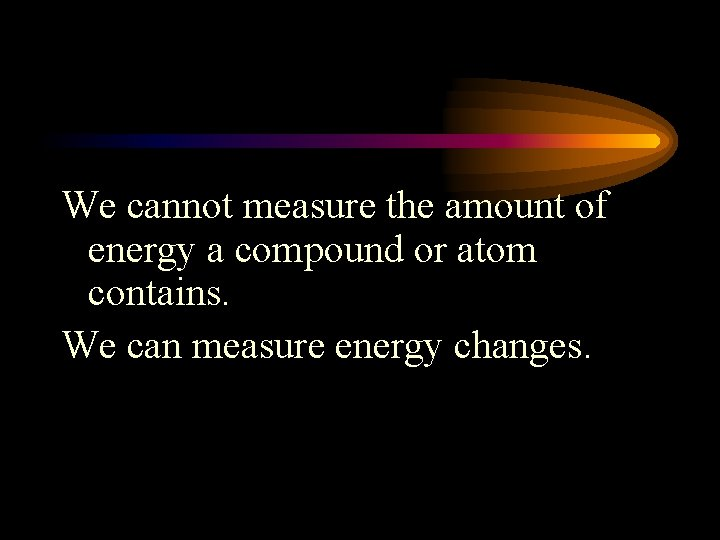We cannot measure the amount of energy a compound or atom contains. We can measure energy changes.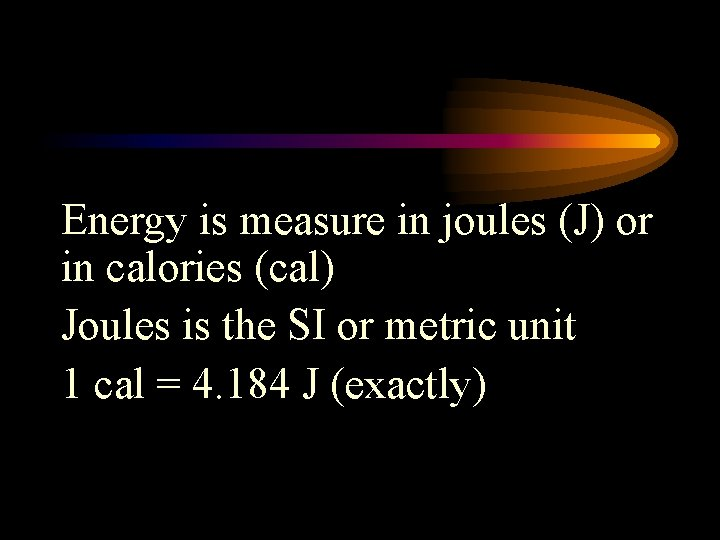Energy is measure in joules (J) or in calories (cal) Joules is the SI or metric unit 1 cal = 4. 184 J (exactly)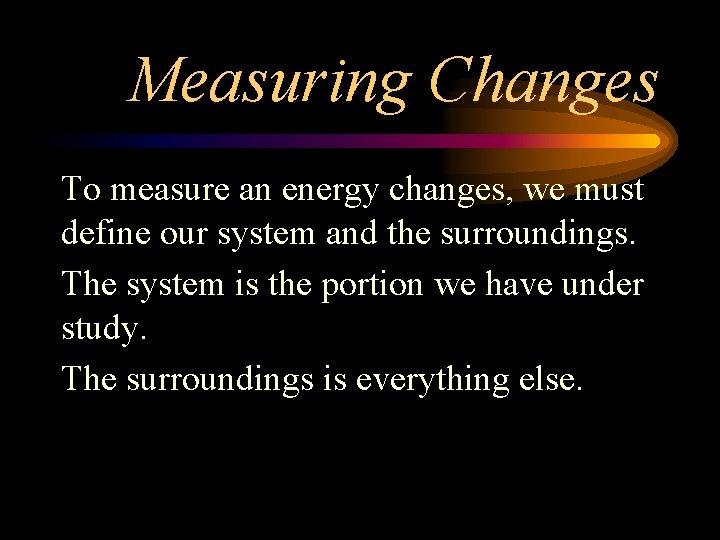Measuring Changes To measure an energy changes, we must define our system and the surroundings. The system is the portion we have under study. The surroundings is everything else.Measuring Change 1 st we can study the changes of internal energy Internal energy is the sum of all the motion in our system and the heat.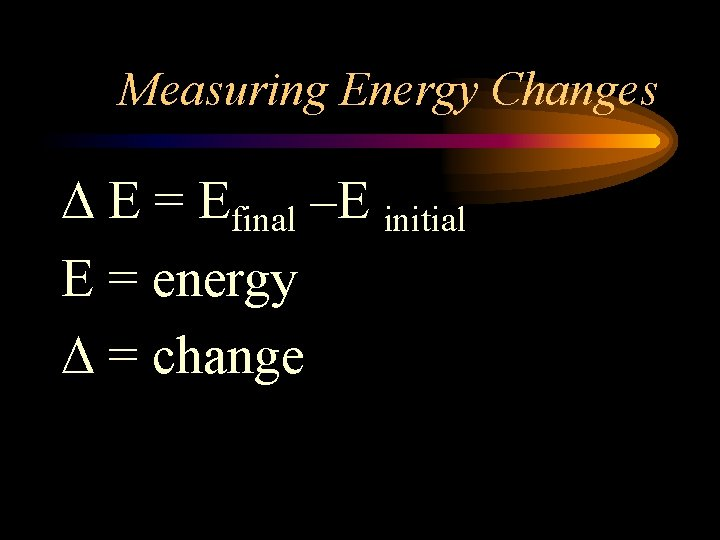Measuring Energy Changes E = Efinal –E initial E = energy = change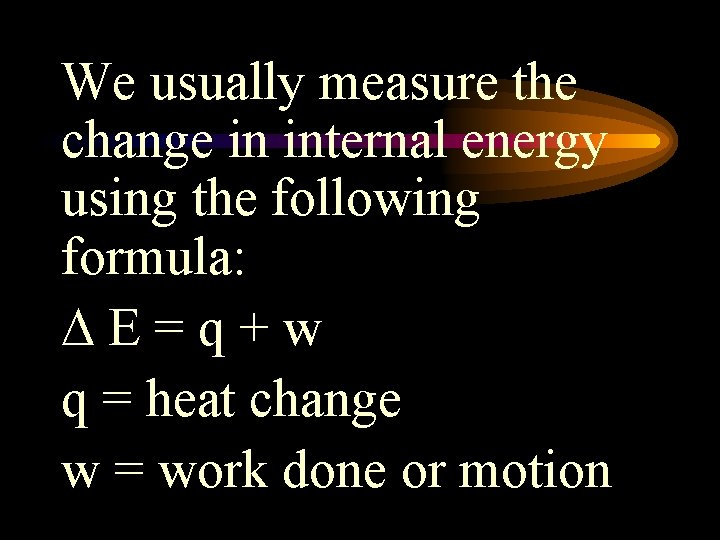We usually measure the change in internal energy using the following formula: E=q+w q = heat change w = work done or motionHeat added to the system has a positive sign. Work done on the system also has a positive sign. A positive sign is a gain in energy. Endothermic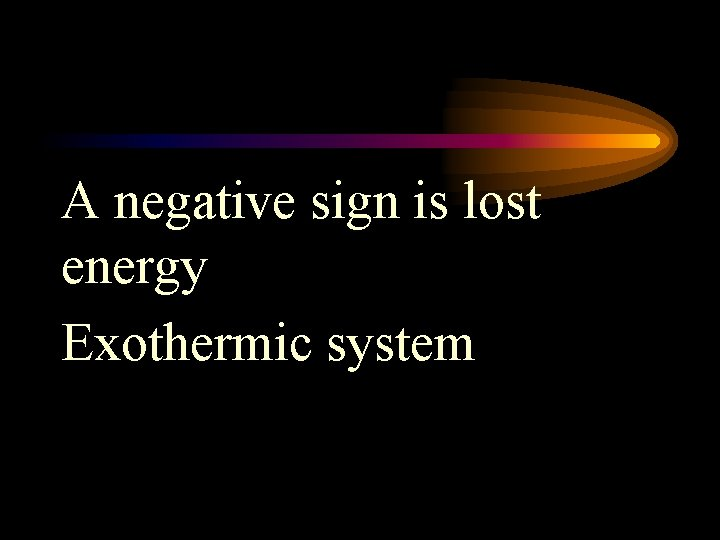A negative sign is lost energy Exothermic systemProblem 11 J of heat were removed, and 45 J of work was done to the system. What is the change in internal energy?Problem 98 J of heat were added and 45 J of work was removed from the system. What is the change in internal energy?State functions A state function is a property of a system which is dependent upon the current conditions. Ex: temperature, pressure, location, etc.State functions The value of a state function does not depend upon its history. Enthalpy is a state function.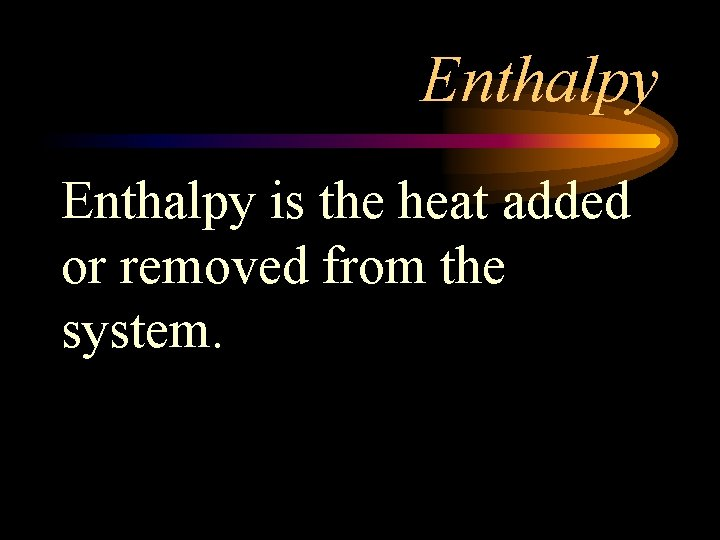Enthalpy is the heat added or removed from the system.Enthalpy is measured as it changes and its symbol is H.Enthalpy H = Hfinal – H initial H > 0 is exothermic H < 0 is endothermic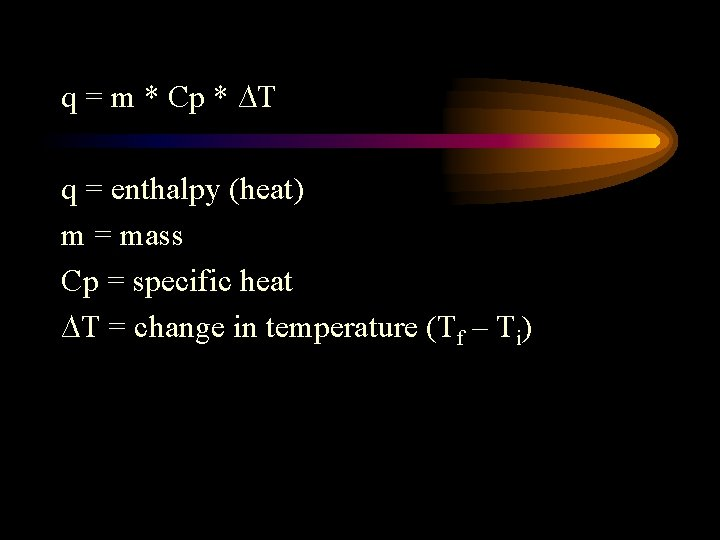q = m * Cp * ∆T q = enthalpy (heat) m = mass Cp = specific heat ∆T = change in temperature (Tf – Ti)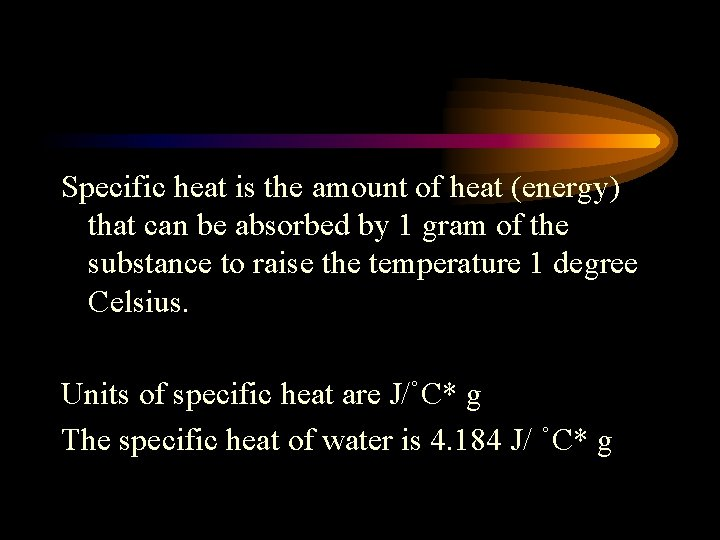Specific heat is the amount of heat (energy) that can be absorbed by 1 gram of the substance to raise the temperature 1 degree Celsius. Units of specific heat are J/˚C* g The specific heat of water is 4. 184 J/ ˚C* g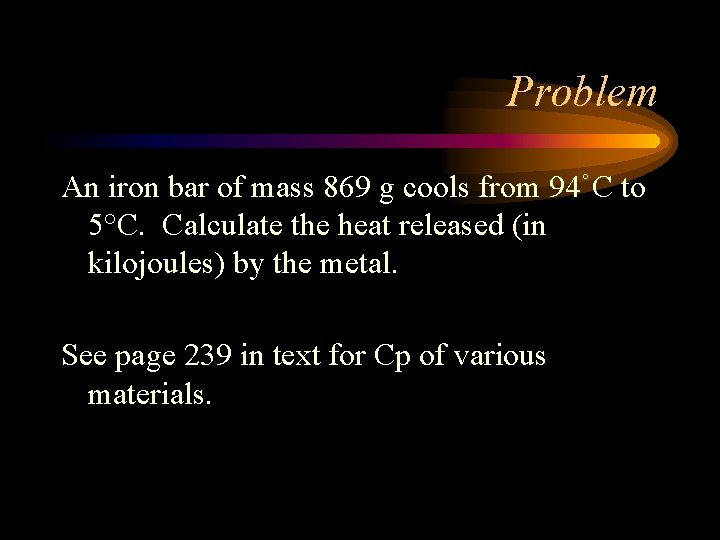Problem An iron bar of mass 869 g cools from 94˚C to 5°C. Calculate the heat released (in kilojoules) by the metal. See page 239 in text for Cp of various materials.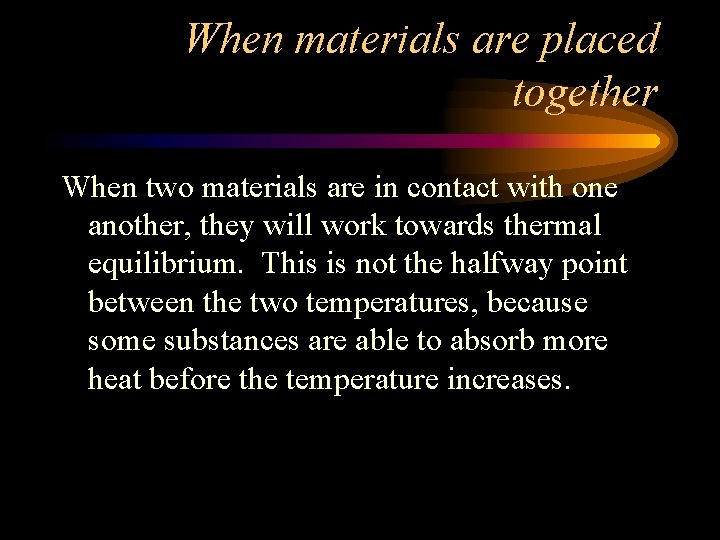When materials are placed together When two materials are in contact with one another, they will work towards thermal equilibrium. This is not the halfway point between the two temperatures, because some substances are able to absorb more heat before the temperature increases.Mathematically The total exchange of heat for the system will equal zero qsystem = qexothermic + qendothermic q exothermic = -qendothermic m 1 Cp 1(Tf-Ti) = -{m 2*Cp 2*(Tf-Ti)} You will have the Tf of 1 will equal the Tf of 2.